University of Bialystok - Central Authentication System You are not logged in | log in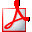Print syllabus

# Algebra II

## General data

 Course ID: 0600-MS1-2ALG2#a Erasmus code / ISCED: 11.102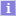/ (0541) MathematicsCourse title: Algebra II Name in Polish: Algebra II Department: Faculty of Mathematics and Informatics Course groups: (in Polish) 3L stac. I st. studia matematyki - przedmioty obowiązkowe ECTS credit allocation (and other scores): 5.00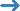view allocation of credits Language: English Type of course: obligatory courses Prerequisites: Algebra I 0600-MS1-2ALG1#aElementary Number Theory 0600-MS1-1ETL#aLinear Algebra II 0600-MS1-1AL2#a Short description: Course objectives: A student can apply the Sylow theorem to describe selected finite groups.A student efficiently uses permutation groups and the classification theorem for finitely generated abelian groups. A student understands the relationship between ideals and algebraic sets. A student understands and can use the Galois theory. Full description: Course profile: academic Form of study: stationary Course type: facultative Academic discipline: Mathematics, field of study in the arts and science: mathematics Year: 2, semester: 4 Prerequisities: Algebra I, Elementary Number Theory, Linear Algebra II lecture 30 h. exercise class 30 h. Verification methods: lectures, exercises, consultations, studying literature, home works, discussions in groups. ECTS credits: 5 Balance of student workload: attending lectures15x2h = 30h attending exercise classes 7x4h + 2h(preliminary teaching) = 30h preparation for classes 7x3h = 21h completing notes after exercises and lectures 7x2h = 14h consultations 5x2h = 10h preparation for control works 2x5h = 10h the final examination: preparation.and take 12h + 3h = 19h Quantitative description Direct interaction with the teacher: 74 h., 2 ECTS Practical exercises: 85 h., 3 ECTS Learning outcomes: Learning outcomes: A student knows that the algebraic structures occurs and are important in various mathematical theories; A student knows the basic concepts of general algebra II and is able to illustrate them on examples (a group action, simple groups, solvable groups, noetherian rings, algebraic sets). A student is able to formulate main theorems of general algebra II (the Sylow theorem, the Galois theorem). A student knows the importance of the Galois theorem in mathematics (i.e. non-solvability by radicals of polynomial equations, non-constructability in geometry). A student knows the contemporary problems of algebra (i.e. the classification of simple groups).K_U17, K_W05, K_W04, K_W01, K_W02 A student can take advantage of the most important general theorem of general algebra II to solve classical exercises. A student can classify finite abelian groups. A student understands problems formulated in the language of abstract algebra and he can formulate problems in this language. A studen can apply euclidean rings to solve diophantine equations.K_U38, K_W02, K_W04 A student can identify a concrete example of application of algebra in reality (i.e. counting of combinatorial objects by the Burnside lemma).K_U29, K_U25, K_W03 A student can present the three famous problems of antiquity and briefly explain the main algebraic ideas which are used in the solution of these problems. K_K02, K_U36, K_W01, K_W03, K_U17 Assessment methods and assessment criteria: The overall form of credit for the course: final exam

## Classes in period "Academic year 2018/2019" (in progress)

 Time span: 2018-10-01 - 2019-06-30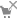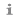Choosen plan division: this week course termsee course schedule Type of class: Class, 30 hoursmore information Lecture, 30 hoursmore information Coordinators: Jarosław Kotowicz Group instructors: (unknown) Students list: (inaccessible to you) Examination: Examination
Course descriptions are protected by copyright.
Copyright by University of Bialystok.